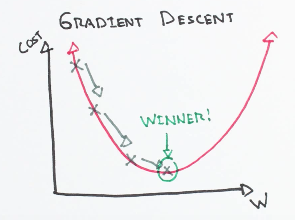/  Deep Learning Interview questions and answers   /  Explain Gradient Descent is going to help to minimize loss functions?## Explain Gradient Descent is going to help to minimize loss functions?

Ans: Gradient descent is an optimization algorithm used to minimize loss function by iteratively moving in the direction of steepest descent that is lowest point in the graph as defined by the negative of the gradient. Our first step is to move towards the down direction specified by negative gradient. After that we have to recalculate the negative gradient and move according to the direction specified by the negative gradient. We continue this process iteratively until e reach to the point global minima.

The size of the step is called learning rate. With high learning rate we can cover more ground in one step but we may not find local minima as the slope of the curve changes randomly. With very low learning rate we can find local minima confidently in the direction specified by negative gradient.

Cost functions or loss functions tells us how well the model is making predictions for a given set of parameters. The slope of the curve tells us how to update parameters to make the model more accurate.

f(m,b)=1N∑i=1n(yi−(mxi+b))2

The gradient can be calculated as: